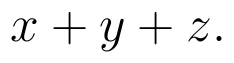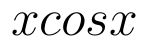Select Page

# CBSE Vector Algebra Maths 12 Science Answers for MCQ in Hindi

CBSE Vector Algebra Maths 12 Science Answers for MCQ in Hindi to enable students to get Answers in a narrative video format for the specific question.

Expert Teacher provides CBSE Vector Algebra Maths 12 Science Answers for MCQ through Video Answers in Hindi language. This video solution will be useful for students to understand how to write an answer in exam in order to score more marks. This teacher uses a narrative style for a question from Vector Algebra not only to explain the proper method of answering question, but deriving right answer too.

Please find the question below and view the Answer in a narrative video format.

Question:

## Similar Questions from CBSE, 12th Science, Maths, Vector Algebra

Question 1 :  Write a unit vector in the direction of the sum of vectorsand(View Answer Video)

Question 2 : Find a vector in the direction of vectorwhich has magnitude 21 units.  (View Answer Video)

Question 3 :  Find the sum of the vectors :(View Answer Video)

Question 4 :  Ifandare two equal vectors, then write the value of(View Answer Video)

Question 5 : Write the position vector of the point which divides the join of points with position vectorsandin the ratio 2:1.  (View Answer Video)

### Inverse Trigonometric Functions

Question 1 :is equal to :

### Relations and Functions

Question 2 : A functionis bijective if and only if, (View Answer Video)

Question 3 : Letbe defined as f(x) = 3x. Choose the correct answer. (View Answer Video)

Question 4 : Letbe defined as. Choose the correct answer. (View Answer Video)

Question 5 : Number of binary sets on the setis, (View Answer Video)

### Continuity and Differentiability

Question 1 : Ifand, find. (View Answer Video)

Question 2 : Differentiate the functionwith respect to x. (View Answer Video)

Question 3 :  Find the second order derivative of the function. (View Answer Video)

Question 4 :  Find the second order derivative of the function. (View Answer Video)

Question 5 : Differentiate the functionwith respect to x. (View Answer Video)